Year 10+ Lines and Angles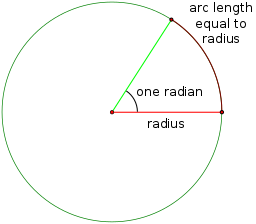As you start to do more complicated mathematics, you will be introduced to the radian as a unit for measuring angles. A radian is the angle formed at the centre of a circle by an arc with the same length as the radius of the circle. Mathematicians like radians for a number of reasons:

1. It is a measurement based on the radius of the circle. Degrees really have nothing to do with the circle.
2. As $x$ gets close to zero, $\sin (x)$ and $\tan (x)$ get close to $x$.
3. Calculus works much more nicely with radians than with degrees. We don't have to multiply by pesky constants.

What Does One Radian Look Like?

So that you can see what one radian looks like, here's an angle that measures 1 radian. 1 radian works out to be $57.2958\dots^\circ$.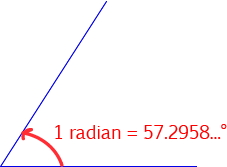Why Such a Funny Number?

Remember that one radian is the angle at the centre of a circle formed by an arc that has the same length as the radius of a circle? Well, the circumference of a circle is given by $2\pi$ times its radius. So, a full revolution is equal to $2\pi \text{ radians}$. In other words, $360^\circ = 2 \pi \text{ radians}$, and so $1 \text{ radian} = \dfrac{360}{2\pi} = \dfrac{180}{\pi}^\circ = 57.2958\dots^\circ$.

Since there are $2\pi$ radians in a whole circle, there are $\pi \text{ radians}$ in a semicircle. This tells us that $\pi \text{ radians} = 180^\circ$, as shown in the picture below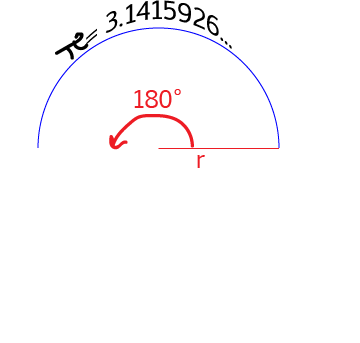The Sizes of Some other Common Angles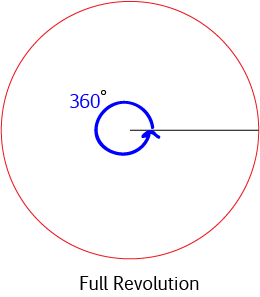A full turn (a full revolution) is $360^\circ$. This is also the angle at the centre of a whole circle. So, a full turn is equal to $2\pi \text{ radians}$.A straight angle (a half turn) is $180^\circ$. This is the angle at the centre of a semicircle, so a straight angle is equal to $\pi \text{ radians}$.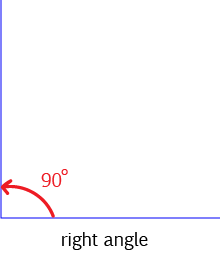A right angle (also known as a quarter turn) is $90^\circ$. This is also the angle at the centre of a quarter circle, so a right angle is equal to $\dfrac{\pi}{2} \text{ radians}$.

In general, to convert from radians to degrees we multiply the angle in radians by $180^\circ$ and divide it by $\pi$.

To convert from degrees to radians, we multiply the angle in degrees by $\pi$ and divide it by $180$.

The table below gives a list of some common angles in degrees, and their equivalents in radians.

$0^\circ$ $0$ $0$
$30^\circ$ $\dfrac{\pi}{6}$ $0.524$
$45^\circ$ $\dfrac{\pi}{4}$ $0.785$
$60^\circ$ $\dfrac{\pi}{3}$ $1.047$
$90^\circ$ $\dfrac{\pi}{2}$ $1.571$
$120^\circ$ $\dfrac{2\pi}{3}$ $2.094$
$135^\circ$ $\dfrac{3\pi}{4}$ $2.356$
$150^\circ$ $\dfrac{5\pi}{6}$ $2.618$
$180^\circ$ $\pi$ $3.142$
$270^\circ$ $\dfrac{3\pi}{2}$ $4.712$
$360^\circ$ $2\pi$ $6.283$

Conclusion

Description

In this mini book, you will learn about

1. Alternate angles
2. Cointerior angles
4. Vertically Opposite Angles

and several other topics related to lines and angles.

Audience

Year 10 or higher

Learning Objectives

To learn the basics of Lines and Angles stream of Geometry

Author: Subject Coach